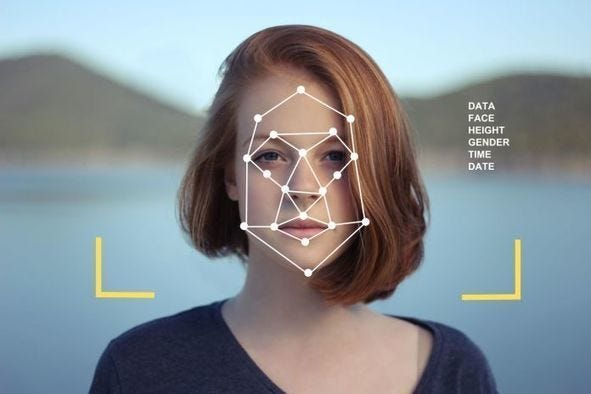# Introduction to Convolutional Neural Networks

Original article was published on Deep Learning on Medium# Introduction to Convolutional Neural Networks

Convolutional Neural Networks are a part of Deep Learning which is employed in image recognition, image classification, object detection, etc. These take an image as input, processes it, and classify it under certain categories.

Here every image is passed through a series of filters, pooling, flattening, and a fully connected layer and apply as the softmax activation function to classify an object with probabilistic values between 0 and 1.

The main reason for applying softmax function is that, as an example, if we are classifying a cat and dog, and let’s say we got a probability that the image is a dog as 0.80 and cat as 0.35. It doesn’t sum to 1 and doesn’t make sense. To overcome it we use softmax function what it does is, It takes the values from the last layer of the hidden layer and then scales the values in between 0 and 1 and make sure it sums to 1.

# How CNN works

## 1.Convolution Layer

It is the first layer to extract features from the image. It keeps the relation between pixels by learning image features using small squares of input data. It extracts features such as edges, corners from the input image.

It performs a dot product between the two matrices, one is the kernel and the other is the portion of the image. The kernel can be of any size but in the visualization shown below the kernel is of size 3 by 3 matrix. It moves from left to right till the end of the image and if any values match with the kernel values, that count is added in the feature detector. If anyone value matches we place 1 at the respective position and if any two values are matched with the kernel we place 2 in the feature detector i.e in the resultant matrix. The representation can be shown below

Stride: It is the number of pixels by which we slide our filter matrix over the input matrix. When the stride is 1 then we move the filters one pixel at a time.

Padding is adding zeros so as to fit it for the stride.

## Relu Layer

We apply a Rectifier function to the Feature Map i.e the Convolved Feature to add Non-Linearity to our image. The reason why we apply non-linearity is, Initially the images are highly non-linear in nature but when we apply Convolution to create Feature Maps, there is a risk that it might create something linear and erases non-linearity. So to make image non-linear we apply rectifier function.

## 2.Max Pooling

In this layer, the dimensionality of the feature map gets reduced by 75% keeping important information. pooling can be of different types:

• Max Pooling
• Average Pooling
• Sum Pooling

Mostly we use Max pooling, pooling won’t only reduce the size by 75% but also prevents overfitting and helps us a lot in terms of processing.

## 3.Flattening

It breaks the spatial structure of the data and transforms your two-dimensional data into one dimensional. This is done to feed the output of CNN to the fully connected network(to classify features learned by CNN) or to feed output to the softmax function to get the probability.